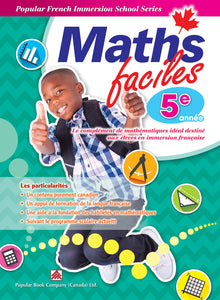# Popular French Immersion School Series: Maths faciles Grade 5

• \$14.95

Maths faciles is a math workbook specially designed for French immersion students. It adopts a progressive approach to help students understand math concepts and master the skills step by step. The fun and easy activities and exercises are preceded by examples and illustrations so that children can enjoy doing them and develop an interest in learning math.

Inside Maths faciles Grade 5 workbook:

Mathematics worksheets cover number patterns, whole numbers, addition, subtraction, multiplication, division, polygons, angles, nets, decimals, data management, time, distance, money, capacity, volume, mass, coordinates, transformations, fractions, multiplication of decimals, division of decimals, length, perimeter, area, patterning with numbers, patterning with shapes, and probability.